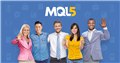# Why this EA code does not work properly?

Here is the code, which I have written:

```//+------------------------------------------------------------------+
//|                                           EMA_12_26_crossing.mq4 |
//|                                  Copyright 2023, MetaQuotes Ltd. |
//|                                             https://www.mql5.com |
//+------------------------------------------------------------------+
#property version   "1.00"
#property strict
//+------------------------------------------------------------------+
//| Expert initialization function                                   |
//+------------------------------------------------------------------+
extern datetime BeginTime = D'2023.05.25 17:01:00'; // trading should begin at this time; only one cross up and one cross down of EMA12 and EMA28 should be traded afterwards
extern double BaseATRMultiplier = 3; // these are not used in current probe version
extern double StopATRMultiplier = 1.15; // there are not used in current probe version
extern double ProfitATRMultiplier = 2.05; // there are not used in current probe version

int OnInit()
{
return(INIT_SUCCEEDED);
}
//+------------------------------------------------------------------+
//| Expert deinitialization function                                 |
//+------------------------------------------------------------------+
void OnDeinit(const int reason)
{
//---
}
//+------------------------------------------------------------------+
//| Expert tick function                                             |
//+------------------------------------------------------------------+

// This expert advisor should only be started when the EMA12 is below the EMA28; an opposite expert advisor should be written and used for the other case

void OnTick()
{

static bool SignalForBuy = false; // this is a flag to track if there has already been and upward EMA crossing; we use this to make sure we only trade one such crossing
static bool SignalForSell = false; // this is a flag to track if there has already been and downward EMA crossing; we use this to make sure we only trade one such crossing
double ExponentialMovingAverage12;
double ExponentialMovingAverage28;
static datetime OneMinuteCandleTime = BeginTime;

if (TimeCurrent() >= BeginTime)

{

if(OneMinuteCandleTime != iTime(Symbol(),PERIOD_M1,0)) // new candle on the 1 minute timeframe

{

OneMinuteCandleTime = iTime(Symbol(),PERIOD_M1,0);

ExponentialMovingAverage12 = NormalizeDouble(iMA(NULL,0,12,0,MODE_EMA,PRICE_CLOSE,1),5);
ExponentialMovingAverage12 = NormalizeDouble(iMA(NULL,0,12,0,MODE_EMA,PRICE_CLOSE,2),5);
ExponentialMovingAverage28 = NormalizeDouble(iMA(NULL,0,28,0,MODE_EMA,PRICE_CLOSE,1),5);
ExponentialMovingAverage28 = NormalizeDouble(iMA(NULL,0,28,0,MODE_EMA,PRICE_CLOSE,2),5);

if (SignalForBuy == false) // if there has't been an upward EMA crossing, we will trade; we only want to trade the first upward crossing

{

if (ExponentialMovingAverage12 - ExponentialMovingAverage28 < 0 && ExponentialMovingAverage12 - ExponentialMovingAverage28 >= 0 )

{

SignalForBuy = true;  // we set this to "true", so that only one upward EMA crossing is traded

}

}

if (SignalForSell == false) // if there has't been a downward EMA crossing, we will trade; we only want to trade the first upward crossing

{

if ((ExponentialMovingAverage12 - ExponentialMovingAverage28 > 0 && ExponentialMovingAverage12 - ExponentialMovingAverage28 <= 0 ) || (ExponentialMovingAverage12 - ExponentialMovingAverage28 == 0 && ExponentialMovingAverage12 - ExponentialMovingAverage28 < 0 ))

{

SignalForSell = true; // we set this to "true", so that only one downward EMA crossing is traded
Alert("We will SELL at this time");

}

}

}

}

}
```Discover new MetaTrader 5 opportunities with MQL5 community and services
• 2023.05.25
• www.mql5.com

mq4...oh sorry i can't help , I have forgotten all about mql4 ....

1. Balint Rubovszky: I have written an MQL4 Expert Advisor code:

Why did you post your MT4 question in the MT5 EA section instead of the MQL4 section, (bottom of the Root page)?
General rules and best pratices of the Forum. - General - MQL5 programming forum? (2017)
Next time, post in the correct place. The moderators will likely move this thread there soon.

2. Balint Rubovszky: When EMA12 gets = EMA28, i
`ExponentialMovingAverage12 - ExponentialMovingAverage28 == 0`

Doubles are rarely equal. Understand the links in:
The == operand. - MQL4 programming forum #2 (2013)

William Roeder #:
1. Why did you post your MT4 question in the MT5 EA section instead of the MQL4 section, (bottom of the Root page)?
General rules and best pratices of the Forum. - General - MQL5 programming forum? (2017)
Next time, post in the correct place. The moderators will likely move this thread there soon.

2. Doubles are rarely equal. Understand the links in:
The == operand. - MQL4 programming forum #2 (2013)

Sorry for not posting at the right place. These doubles are rounded to 5 digits, and if you check the "GDAX EMA Cross[26,12] by stefano89" indicator on TradingView for GBPUSD, sometimes, the values are equal. I wish to mimic the operation of that indicator.

Balint Rubovszky:
double ExponentialMovingAverage12;     double ExponentialMovingAverage28;

You declared the wrong array number.

``` double ExponentialMovingAverage12;
``` double ExponentialMovingAverage12;